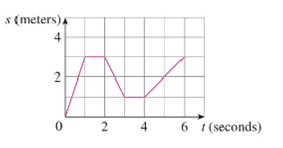Chapter 2.7, Problem 11E

Chapter
Section
Textbook Problem

(a) A particle starts by moving to the right along a horizontal line; the graph of its position function is shown in the figure. When is the particle moving to the right? Moving to the left? Standing still?(b) Draw a graph of the veloc.ity function.(a)

To determine

To state: The position at which the particle is moving to the right, moving to the left and standing still on the time intervals.

Explanation

Formula used:

The average velocity over the time interval [a,a+h] is,

Average velocity=displacementtime

=f(a+h)f(a)(a+h)a (1)

Note 1: The average velocity is same as the instantaneous velocity when the particle is moving along a straight line.

Note 2: The particle is moving to the right when the velocity is positive.

Note 3: The particle is moving to the left when the velocity is negative.

Note 4: The particle is standing still when the velocity is zero.

Calculation:

At the time interval (0, 1):

From the given graph, it is observed that the straight line is passing through the points (0, 0) and (1,3).

Substitute (0, 0) for (a,f(a)) and (1,3) for (a+h,f(a+h)) in equation (1),

Average velocity=3010=31=3

Thus, the average velocity of the particle moving along a straight line is 3 m/s.

By Note 1, the velocity of the particle on the time interval (0, 1) is 3 m/s.

By Note 2, the particle is moving to the right on the time interval (0, 1) since the velocity is positive.

Thus, the particle is moving to the right when s(t) is increasing on the interval (0, 1).

At the time interval (1, 2):

From the given graph, it is observed that the straight line is passing through the points (1, 3) and (2,3).

Substitute (1, 3) for (a,f(a)) and (2,3) for (a+h,f(a+h)) in equation (1),

Average velocity=3321=01=0

Thus, the average velocity of the particle moving along a straight line is 0 m/s.

By Note 1, the velocity of the particle on the time interval (1, 2) is 0 m/s.

By Note 4, the particle is standing still on the time interval (1, 2), since the velocity is zero.

Thus, the particle is standing still when s(t) is constant on the interval (1, 2).

At the time interval (2, 3):

From the given graph, it is observed that the straight line is passing through the points (2, 3) and (3,1)

(b)

To determine

To sketch: The graph of the velocity function.

Still sussing out bartleby?

Check out a sample textbook solution.

See a sample solution

The Solution to Your Study Problems

Bartleby provides explanations to thousands of textbook problems written by our experts, many with advanced degrees!

Get Started

In Exercises 7-28, perform the indicated operations and simplify each expression. 16. x1x+2x+3x21

Applied Calculus for the Managerial, Life, and Social Sciences: A Brief Approach

Find the derivative of the function. F(t) = (3t 1)4(2t + 1)3

Single Variable Calculus: Early Transcendentals, Volume I

A sample of n = 9 scores has X = 108. What is the sample mean?

Essentials of Statistics for The Behavioral Sciences (MindTap Course List)

Explain the terms (a) scatter diagram (b) least-squares line.

Finite Mathematics for the Managerial, Life, and Social Sciences

Find for x = 3t2 + 1, y = t6 + 6t5. t4 + 5t3 4t3 + 15t2

Study Guide for Stewart's Multivariable Calculus, 8th

Sometimes, Always, or Never: If limxaf(x) and f(a) both exist, then f is continuous at a.

Study Guide for Stewart's Single Variable Calculus: Early Transcendentals, 8th